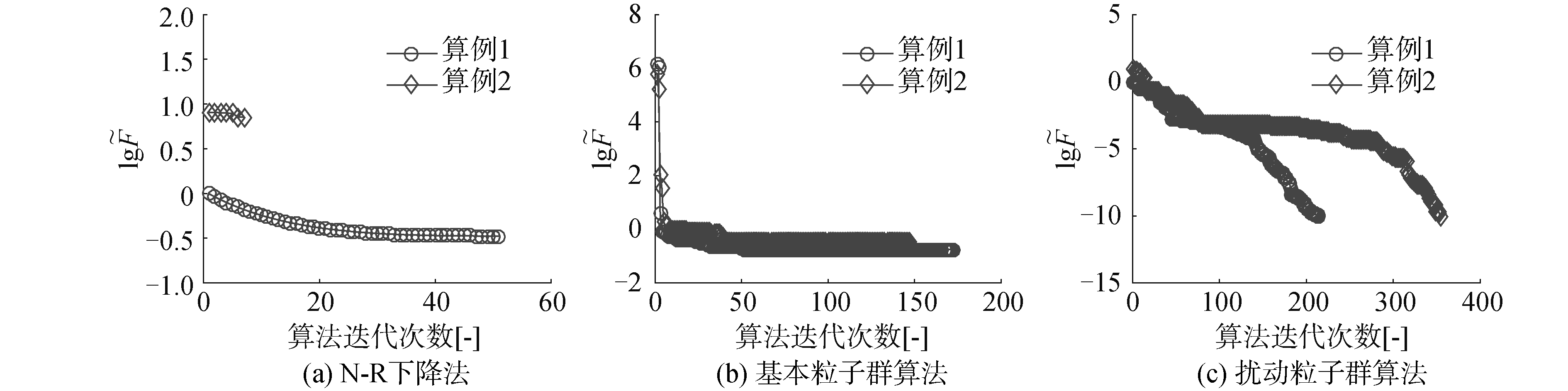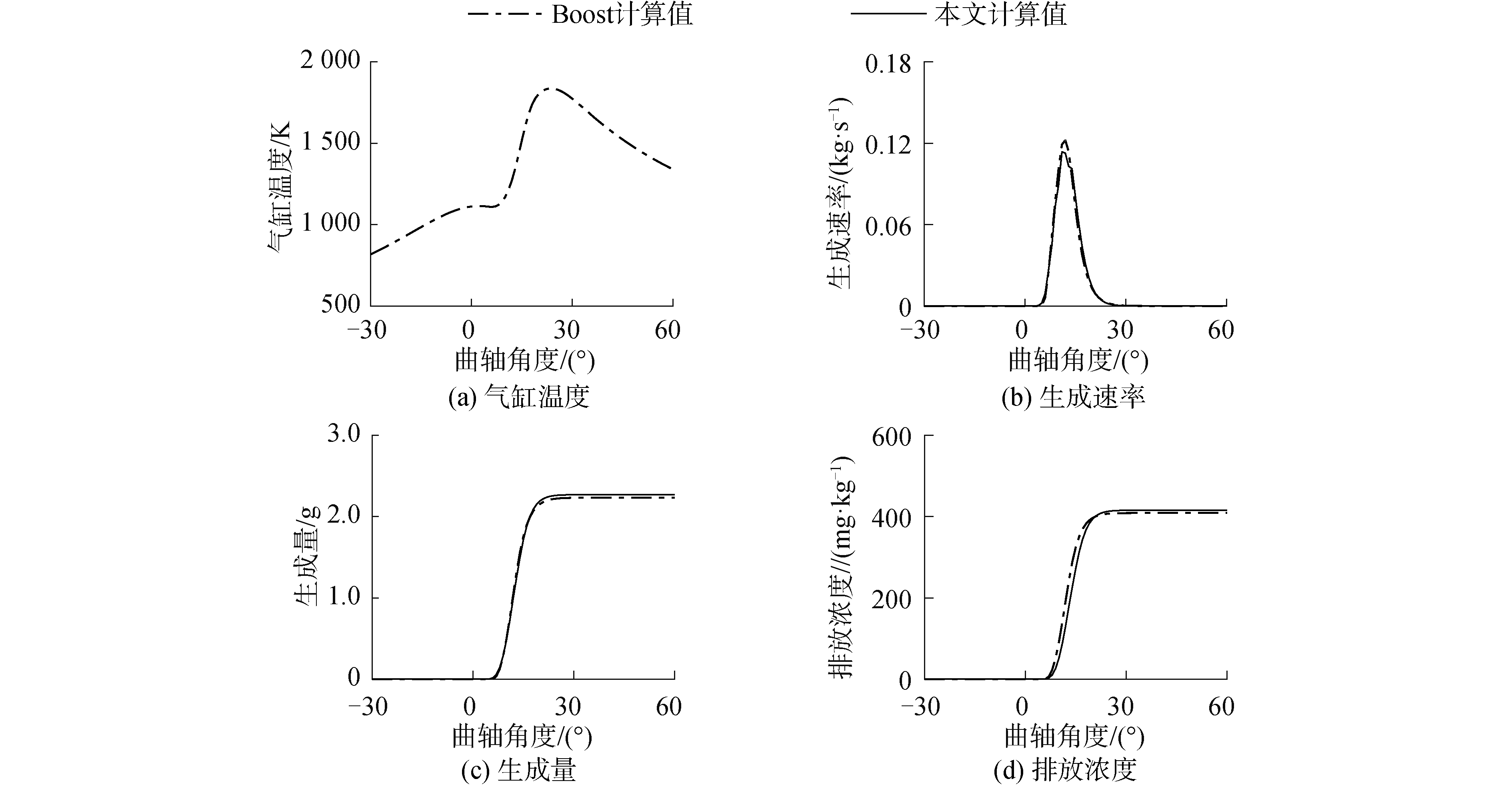﻿ 柴油机燃烧产物的扰动粒子群求解方法
«上一篇文章快速检索 高级检索

 哈尔滨工程大学学报2019, Vol. 40Issue (9): 1636-1641  DOI: 10.11990/jheu.2018030150

### 引用本文TANG Yuanyuan, ZHANG Jundong, LU Daoyi, et al. Perturbed particle swarm optimization for determining diesel engine combustion products[J]. Journal of Harbin Engineering University, 2019, 40(9), 1636-1641. DOI: 10.11990/jheu.201803015.### 文章历史

1. 大连海事大学 轮机工程学院, 辽宁 大连 116026;
2. 中船动力研究院有限公司 上海 200120

Perturbed particle swarm optimization for determining diesel engine combustion products
TANG Yuanyuan 1, ZHANG Jundong 1, LU Daoyi 1, ZENG Hong 1, XIA Yu 2
1. College of Marine Engineering, Dalian Maritime University, Dalian 116026, China;
2. China Shipbuilding Power Engineering Institute Co., Ltd., Shanghai 200120, China
Abstract: To solve the difficult problem of equilibrium concentration of combustion products in internal combustion engines, the problem is transformed into a nonlinear equation problem using the equilibrium constant method. These nonlinear equations are transformed into an unconstrained optimization problem using a two-norm format to obtain a better convergence and avoid matrix singularity. Based on the basic particle swarm optimization (PSO), the initial value of a particle is modified from a random free distribution to a distribution near a given initial value to speed up the convergence. The search path perturbation is added to the search path to enhance the global convergence capability. The perturbed PSO can be successfully used to predict the formation of nitrogen oxides in internal combustion engines. The convergence accuracy can reach 10-10 order of magnitude. Moreover, the implementation is simple, and the global convergence ability is strong. Compared with the Newton-Raphson algorithm, basic PSO algorithm, and STANJAN algorithm, the perturbed PSO exhibited higher precision and better stability.
Keywords: diesel engine    Newton-Raphson    combustion products    particle swarm optimization    nitrogen oxides    chemical equilibrium    STANJAN algorithm    equilibrium constant

1 STANJAN算法

STANJAN算法使用最小化Gibbs自由焓的方法计算物质化学反应平衡时的浓度。在STANJAN算法中假设系统包含s种物质，所有物质由a种元素构成。一个物质j分子中具有原子i的个数为nij，物质j在相m中的摩尔分数为xj，相m中的所有物质的物质的量为Nm，则根据原子守恒和摩尔分数的定义有:

 $\sum\limits_{j = 1}^s {{n_{ij}}} {\bar N_j}{x_j} = {P_i}, \quad i = 1, 2, \cdots , a$ (1)
 $\sum\limits_{j = 1}^s {{x_j}} = 1$ (2)

 ${x_j} = \exp \left( { - {{\tilde g}_j} + \sum\limits_{i = 1}^s {{\lambda _i}} {n_{ij}}} \right), \quad j = 1, 2, \cdots , s$ (3)

2 扰动粒子群算法 2.1 优化目标

 $F(X) = Y$ (4)

 $\bar F(X) = F(X) - Y$ (5)

 $\tilde F = {\left\| {\bar F} \right\|_2} = \sqrt {{{\left( {{{\bar f}_1}} \right)}^2} + l + {{\left( {{{\bar f}_n}} \right)}^2}} \ge 0$ (6)

 $\min \tilde F \Leftrightarrow \bar F(X) = 0 \Leftrightarrow F(X) = Y$ (7)

2.2 粒子群算法

 $\left\{ {\begin{array}{*{20}{l}} {{\mathit{\boldsymbol{v}}_{k + 1}} = \mathit{\boldsymbol{\omega }}{\mathit{\boldsymbol{v}}_k} + {\varphi _1}{r_1}\left( {{\mathit{\boldsymbol{x}}_k} - {\mathit{\boldsymbol{x}}_p}} \right) + {\varphi _2}{r_2}\left( {{\mathit{\boldsymbol{x}}_k} - {\mathit{\boldsymbol{x}}_g}} \right)}\\ {{\mathit{\boldsymbol{x}}_{k + 1}} = {\mathit{\boldsymbol{x}}_k} + {\mathit{\boldsymbol{v}}_k}} \end{array}} \right.$ (8)

2.3 扰动粒子群算法

 ${x_0} = x_0^* + 0.2\left( {{N_{{\rm{rnd}}}} - 0.5} \right)$ (9)

 ${x_i} = {x_i} + 2a\left( {{N_{{\rm{rnd}}}} - 0.5} \right){x_i}$ (10)
3 算法性能分析表 1 算例1中燃烧产物平衡时的摩尔分数 Table 1 The mole fractions of combustion products at chemical equilibrium in case 1表 2 算例2中燃烧产物平衡时的摩尔分数 Table 2 The mole fractions of combustion products at chemical equilibrium in case 2

 $\rho (a, b) = \sqrt {{{\left( {\ln {a_1}/{b_1}} \right)}^2} + \cdots + {{\left( {\ln {a_n}/{b_n}} \right)}^2}}$ (11)表 3 算例1中算法性能对比 Table 3 The comparasion of algorithms in case 1表 4 算例2中算法性能对比 Table 4 The comparasion of algorithms in case 2Download: 图 1 Newton-Raphson(N-R)下降法、基本粒子群算法、扰动粒子群算法的收敛过程 Fig. 1 The convergence of Newton-Raphson (N-R) downhill method, basic PSO, and perturbed PSO
4 氮氧化物生成预测Download: 图 2 缸内一氧化氮生成预测 Fig. 2 The prediction of nitric oxide in cylinder
5 结论

1) 扰动粒子群算法可以准确的计算出燃烧产物的平衡浓度。扰动粒子群算法实现简单，与迭代法相比具有更强的寻优能力，与基本粒子群算法相比具有更强的全局寻优能力，能够有效的避免陷入局部最优值。

2) STANJAN算法寻优过程采用的是最速下降法和N-R迭代法，其寻优能力与N-R迭代法相当。STANJAN算法增加了许多保证算法收敛的技术，算法稳定性比单纯使用N-R迭代法要好。

3) 使用最小化Gibbs自由焓的方法和使用平衡常数法求解化学反应平衡浓度的结果基本一致。在燃烧反应中考虑的物质种类较多时，使用最小化Gibbs自由焓可以得到更小的方程组，求解过程相对平衡常数法更为简单。

  WEI Mingrui, THANH SA N, TURKSON R F, et al. Water injection for higher engine performance and lower emissions[J]. Journal of the energy institute, 2017, 90(2): 285-299. DOI:10.1016/j.joei.2015.12.003 (0)  VELLAIYAN S, AMIRTHAGADESWARAN K S. The role of water-in-diesel emulsion and its additives on diesel engine performance and emission levels:a retrospective review[J]. Alexandria engineering journal, 2016, 55(3): 2463-2472. DOI:10.1016/j.aej.2016.07.021 (0)  THANGARAJA J, KANNAN C. Effect of exhaust gas recirculation on advanced diesel combustion and alternate fuels-a review[J]. Applied energy, 2016, 180: 169-184. DOI:10.1016/j.apenergy.2016.07.096 (0)  MOHAN B, YANG Wenming, CHOU S K. Fuel injection strategies for performance improvement and emissions reduction in compression ignition engines-a review[J]. Renewable and sustainable energy reviews, 2013, 28: 664-676. DOI:10.1016/j.rser.2013.08.051 (0)  AGARWAL A K, SINGH A P, MAURYA R K. Evolution, challenges and path forward for low temperature combustion engines[J]. Progress in energy and combustion science, 2017, 61: 1-56. DOI:10.1016/j.pecs.2017.02.001 (0)  GURUSAMY P, GANDHI U, MANGALANATHAN U, et al. Measurement of diesel exhaust fluid concentration in urea-SCR after-treatment system[J]. International journal of precision engineering and manufacturing, 2017, 18(8): 1085-1092. DOI:10.1007/s12541-017-0127-z (0)  牛晓晓, 王银燕, 王正祥, 等. 柴油机性能及排放预测高效试验设计应用研究[J]. 应用科技, 2018, 45(05): 95-101. NIU Xiaoxiao, WANG Yinyan, WANG Zhengxiang, et al. Application of high-efficiency experimental design method for predicting the performance and emission of diesel engine[J]. Applied science and technology, 2018, 45(05): 95-101. (0)  ZELDOVICH J. The oxidation of nitrogen in combustion and explosions[J]. Acta physicochim, 1946, 21(4): 577-628. (0)  LAVOIE G A, HEYWOOD J B, KECK J C. Experimental and theoretical study of nitric oxide formation in internal combustion engines[J]. Combustion science and technology, 1970, 1(4): 313-326. DOI:10.1080/00102206908952211 (0)  GORDON S, MCBRIDE B J. Computer program for calculation of complex chemical equilibrium compositions, rocket performance, incident and reflected shocks, and chapman-jouguet detonations[R]. Washington, D.C.: National Aeronautics and Space Administration, 1976. (0)  REYNOLDS W C. The element potential method for chemical equilibrium analysis: implementation in the interactive program STANJAN[R]. California: Stanford University, Department of Mechanical Engineering, 1986. (0)  POPE S B. Gibbs function continuation for the stable computation of chemical equilibrium[J]. Combustion and flame, 2004, 139(3): 222-226. DOI:10.1016/j.combustflame.2004.07.008 (0)  RAKOPOULOS C D, HOUNTALAS D T, TZANOS E I, et al. A fast algorithm for calculating the composition of diesel combustion products using 11 species chemical equilibrium scheme[J]. Advances in engineering software, 1994, 19(2): 109-119. DOI:10.1016/0965-9978(94)90064-7 (0)  周松, 马强, 李晓波. 内燃机一氧化氮生成机理及计算研究[J]. 哈尔滨工程大学学报, 1997, 18(4): 25-30. ZHOU Song, MA Qiang, LI Xiaobo. The study of formation mechanism and calculation of nitric oxide in internal combustion engine[J]. Journal of Harbin Engineering University, 1997, 18(4): 25-30. (0)  周松, 马强, 熊承刚. 内燃机燃烧产物平衡浓度及化学反应平衡常数的计算[J]. 哈尔滨工程大学学报, 1997, 18(5): 85-89. ZHOU Song, MA Qiang, XIONG Chengang. Calculation of equilibrium concentration of combustion products and equilibrium constant of chemical reactions in internal combustion engine[J]. Journal of Harbin Engineering University, 1997, 18(5): 85-89. (0)  ANETOR L, ODETUNDE C, OSAKUE E E. Computational analysis of the extended Zeldovich mechanism[J]. Arabian journal for science and engineering, 2014, 39(11): 8287-8305. DOI:10.1007/s13369-014-1398-7 (0)  ONUH EI, INAMBAO FL. An evaluation of neat biodiesel/diesel performance, emission pattern of NOx and CO in compression ignition engine[J]. International journal of global warming, 2018, 14: 21-39. (0)  TANG Yuanyuan, ZHANG Jundong, GAN Huibing, et al. Development of a real-time two-stroke marine diesel engine model with in-cylinder pressure prediction capability[J]. Applied energy, 2017, 194: 55-70. DOI:10.1016/j.apenergy.2017.03.015 (0)  唐元元, 张均东, 贾宝柱, 等. 气缸体对船舶柴油机模型精度的影响[J]. 哈尔滨工程大学学报, 2017, 38(12): 1836-1843. TANG Yuanyuan, ZHANG Jundong, JIA Baozhu, et al. The effect of cylinder body on the accuracy of marine diesel engine model[J]. Journal of Harbin Engineering University, 2017, 38(12): 1836-1843. (0)